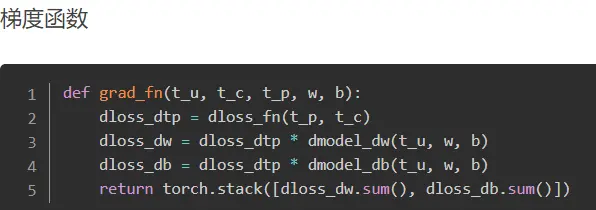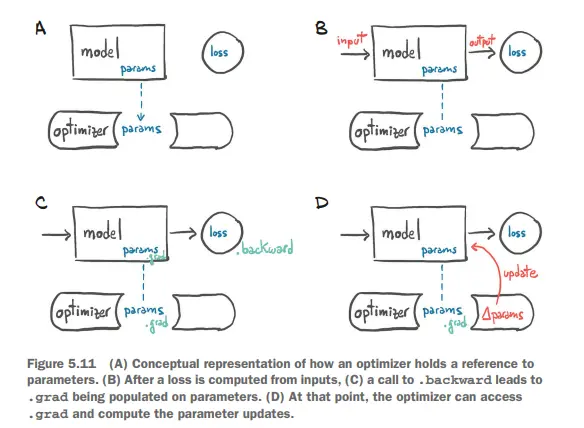# 8 从0开始学PyTorch | PyTorch中自动计算梯度、使用优化器

#### 自动计算梯度

params = torch.tensor([1.0, 0.0])

%matplotlib inline
import numpy as np
import torch
torch.set_printoptions(edgeitems=2)

t_c = torch.tensor([0.5, 14.0, 15.0, 28.0, 11.0, 8.0,
3.0, -4.0, 6.0, 13.0, 21.0])
t_u = torch.tensor([35.7, 55.9, 58.2, 81.9, 56.3, 48.9,
33.9, 21.8, 48.4, 60.4, 68.4])
t_un = 0.1 * t_u

def model(t_u, w, b):
return w * t_u + b

def loss_fn(t_p, t_c):
squared_diffs = (t_p - t_c)**2
return squared_diffs.mean()

#唯一改变


loss = loss_fn(model(t_u, *params), t_c)
loss.backward() #对loss进行反向传播

#输出params的梯度看看
outs:tensor([4517.2969,   82.6000])image.png

if params.grad is not None:


def training_loop(n_epochs, learning_rate, params, t_u, t_c):
for epoch in range(1, n_epochs + 1):

t_p = model(t_u, *params)
loss = loss_fn(t_p, t_c)
loss.backward()

if epoch % 500 == 0:
print('Epoch %d, Loss %f' % (epoch, float(loss)))

return params


#### 优化器

dir() 函数不带参数时，返回当前范围内的变量、方法和定义的类型列表；带参数时，返回参数的属性、方法列表。如果参数包含方法dir()，该方法将被调用。如果参数不包含dir()，该方法将最大限度地收集参数信息。

import torch.optim as optim

dir(optim)
outs:
['ASGD',
'LBFGS',
'Optimizer',
'RMSprop',
'Rprop',
'SGD',
'__builtins__',
'__cached__',
'__doc__',
'__file__',
'__name__',
'__package__',
'__path__',
'__spec__',
'_functional',
'_multi_tensor',
'lr_scheduler',
'swa_utils']image.png

params = torch.tensor([1.0, 0.0], requires_grad=True)
learning_rate = 1e-5
optimizer = optim.SGD([params], lr=learning_rate)

t_p = model(t_u, *params)
loss = loss_fn(t_p, t_c)
loss.backward()

optimizer.step() #调用step()方法，就会更新params的值

params
outs:


def training_loop(n_epochs, optimizer, params, t_u, t_c):
for epoch in range(1, n_epochs + 1):
t_p = model(t_u, *params)
loss = loss_fn(t_p, t_c)

loss.backward()
optimizer.step()

if epoch % 500 == 0:
print('Epoch %d, Loss %f' % (epoch, float(loss)))

return params

learning_rate = 1e-2
optimizer = optim.SGD([params], lr=learning_rate)

training_loop(
n_epochs = 5000,
optimizer = optimizer,
params = params,
t_u = t_un,
t_c = t_c)

outs:
Epoch 500, Loss 7.860115
Epoch 1000, Loss 3.828538
Epoch 1500, Loss 3.092191
Epoch 2000, Loss 2.957698
Epoch 2500, Loss 2.933134
Epoch 3000, Loss 2.928648
Epoch 3500, Loss 2.927830
Epoch 4000, Loss 2.927679
Epoch 4500, Loss 2.927652
Epoch 5000, Loss 2.927647


07-111407
05-182753
09-02685
01-171874
09-14959
01-081722
10-20556
03-214万+
10-27494
01-301万+
07-292121
12-111万+
04-292067
12-111648
11-237173

### “相关推荐”对你有帮助么？

•非常没帮助
•没帮助
•一般
•有帮助
•非常有帮助被折叠的  条评论 为什么被折叠?到【灌水乐园】发言¥1 ¥2 ¥4 ¥6 ¥10 ¥20获取中扫码支付点击重新获取扫码支付1.余额是钱包充值的虚拟货币，按照1:1的比例进行支付金额的抵扣。
2.余额无法直接购买下载，可以购买VIP、付费专栏及课程。余额充值